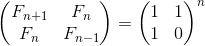# How will you come up with a logn solution?

#1

Comment body goes here.

#2

As you can see the recurrence we get in the solution is

rec(n) = rec(n-1) + rec(n-2)

Does this look familiar?
This is the fibonacci sequence.

One way we can solve the same is by using matrices as shown in the following equationNow to solve for the power n in O(log n) time using binary exponentiation which gives us the desired O(log n) solution.

#3

Use matrix exponentiation